## 2016年5月17日 星期二

### 給新手的C++教學 (上冊) - 9. 函式 (Function)

#include<cstdio>
int main()
{
int n;
scanf("%d",&n);
int a=1,b=1,c=1;
int i=1;
while(i<=2*n)
{
a=a*i;
i=i+1;
}
i=1;
while(i<=n)
{
b=b*i;
i=i+1;
}
i=1;
while(i<=2*n-n)
{
c=c*i;
i=i+1;
}
int first=a/(b*c);
a=1,b=1,c=1,i=1;
while(i<=2*n)
{
a=a*i;
i=i+1;
}
i=1;
while(i<=n-1)
{
b=b*i;
i=i+1;
}
i=1;
while(i<=2*n-(n-1))
{
c=c*i;
i=i+1;
}
int second=a/(b*c);
printf("%d\n",first-second);
return 0;
}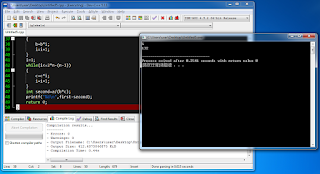當輸入$n=6$時，程式輸出第6個卡特蘭數「132」了！

(其實，程式的「函式」就是數學的「函數」，英文名稱都是「Function」)

int function_name(int a)
{
你的程式碼
return 算出來的答案;
}

#include<cstdio>
int f(int x)
{
return x*x*x+3*x+32;
}
int main()
{
int a;
scanf("%d",&a);
printf("%d\n",f(a));
return 0;
}

int a=1,i=1;
while(i<=n)
{
a=a*i;
i=i+1;
}

#include<cstdio>
int Level(int n)
{
int a=1,i=1;
while(i<=n)
{
a=a*i;
i=i+1;
}
return a;
}
int main()
{
int n;
scanf("%d",&n);
int first=Level(2*n)/(Level(n)*Level(2*n-n));
int second=Level(2*n)/(Level(n+1)*Level(2*n-(n+1)));
printf("%d\n",first-second);
return 0;
}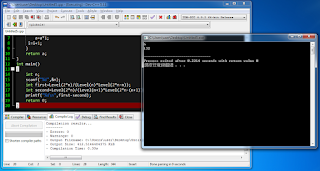當輸入$n=6$時，程式依然是正確地輸出「132」！

#include<cstdio>
int Level(int n)
{
int a=1,i=1;
while(i<=n)
{
a=a*i;
i=i+1;
}
return a;
}
int C(int a,int b)
{
return Level(a)/(Level(b)*Level(a-b));
}
int main()
{
int n;
scanf("%d",&n);
printf("%d\n",C(2*n,n)-C(2*n,n+1));
return 0;
}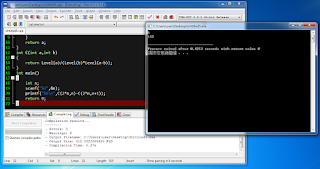當輸入$n=6$時，程式仍然是正確地輸出「132」！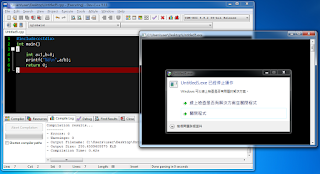我們讓程式執行「除以零」的動作，程式就出錯了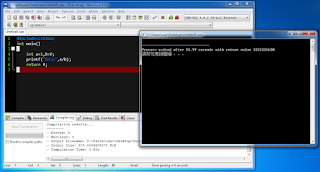程式出錯的方式有很多種，每一種錯誤都會導致程式回傳特定的非零數字 當我們點選「關閉程式」之後，可以看到程式的回傳值 (僅限用Dev-C++寫出來的終端機程式) 例如這個「除以零」的錯誤會得到回傳值「3221225620」

(版權所有 All copyright reserved)

#### 13 則留言:

1.函式裡面麼塞陣列啊?(我是指把陣列當變數)

1.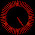嗨～
直接舉個例子好了：
#include<cstdio>
int Sum(int n,int s[])
{
int answer=0;
for(int i=0;i<n;i++)
{
answer=answer+s[i];
}
return answer;
}
int main()
{
int s;
for(int i=0;i<5;i++)
{
s[i]=i;
}
printf("%d\n",Sum(5,s));
return 0;
}

有不懂歡迎再問哦～^_^

2.請問一下
在算卡特蘭數時，為什麼到第7位以後就會溢位呢？
明明第7位是429、第8位是1430，兩個數都沒有很大啊？？

1.因為卡特蘭數的第7項要計算14!(=87178291200)，溢位囉～XD

2.喔喔所以是過程中數字太大，而不是結果太大對吧XD
那有沒有辦法算這些大數的功能呢

3.想請問一下
若程式最後沒有寫return 0
會怎麼樣嗎？？

1.只能說，絕大部分情況下不會怎麼樣。但如果您的程式被另一個程式監控，您的程式執行結果(main函式的回傳值)就會影響到另一個程式的行為(通常0代表正常，非0代表有錯誤)。沒有加return 0好像它也會自動幫你return 0的樣子，不過在C語言就不會囉～而且不一定所有的C++編譯器都有依照規定設計(如自動return 0)

總之
加了return 0保證沒事
不加，也可以，但潛在的風險請自行負責XD

4.想問一下
我寫了C幾取幾的程式
main function裡面
是要先cin a、b才能寫combination嗎？
為什麼不行顛倒呢？

#include <iostream>
using namespace std;

int Level(int n)
{
int i, level = 1;
for (i = 1; i <= n; i++)
level *= i;
return level;
}

int main(int argc, char **argv)
{
int combination, a, b;

cin >> a >> b;
combination = (Level(a) / (Level(b) * Level(a-b)));
cout << combination << endl;
}

1.這該怎麼解釋呢...XD
要先設定好a、b的值，拿a、b來做計算的結果才是正確的呀～

5.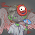我前面n!和2n!的程式碼都和你一樣
但是我把卡特蘭數乘開 沒有用c
變成a/(b*b)*(1-n/(n+1))
但是答案都不對 輸入1變2 輸入2變6 輸入3變20

6.作者已經移除這則留言。

7.小莫大大:
#include<cstdio>
int fl(int x)
{
int times=1,count=1;
while (x>=times)
{
count=count*times;
times+=1;
}
return count;
}
int ka(int a,b)
{
int count;
count=fl(a);
count=count/fl(b);
count=count/fl(a-b);
return count;
}
int main()
{
int n,count,times=1;
printf("到第幾項:");
scanf("%d",&n);
while (n>=times)
{
count=ka(times,times*2)-ka(times*2,times+1);
printf("%d",count);
}
return 0;
}
電腦一直說有問題 請幫我看看

8.我算卡特蘭數的code和上面的範例一樣,但答案都不對
不知道是因為程式的問題還是別的方面